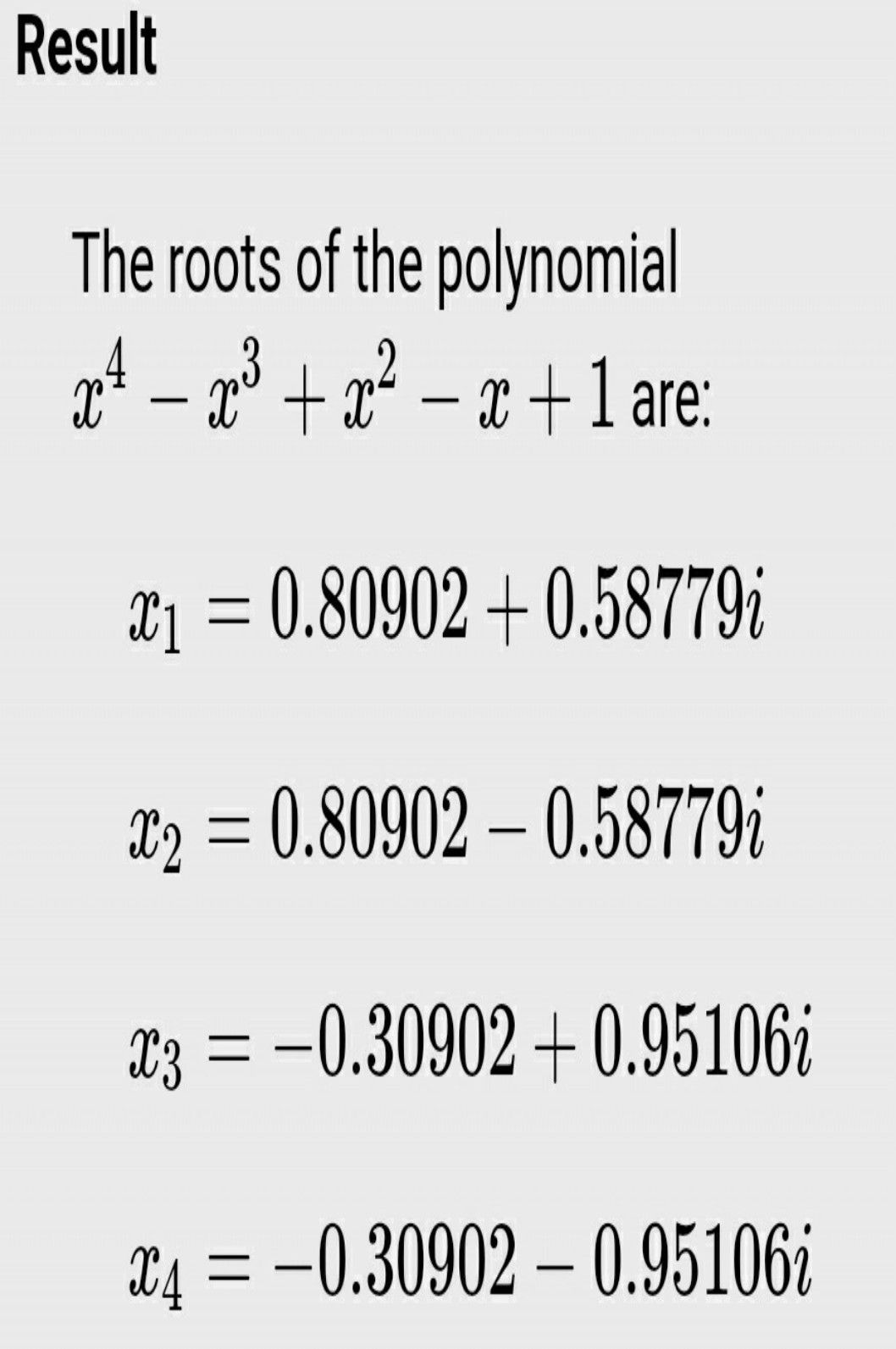Symbol
Problem$6$ $O$ Obtain the roots $0f$ $\left(1\right)$ $x^{4}-x^{3}+x^{2}-x+1=0$ $\left(ii\right)$ $x^{4}+x^{3}+x^{2}+x+1=0$
10th-13th grade
Geometry
SolutionQanda teacher - Chitta
i am giving you answer with in 10 minutesStudent
please do fastlyQanda teacher - Chitta
ok
see i am trying to solve it by calculations but i didn't find any real root so i go through online and i found thisStudent
but sir i need step wise solnQanda teacher - Chitta
ok i know but have tried my best but i didn't get any real root sorry for the inconveniences with me
is there any way to give your coin to you
if you know please tellStudent
I don't know its okQanda teacher - Chitta
ok i will try again
if something is possible then I will give your answer